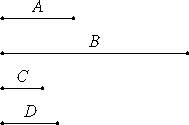# Proposition 17

If a cubic number does not measure a cubic number, then neither does the side measure the side; and, if the side does not measure the side, then neither does the cube measure the cube.

Let the cubic number A not measure the cubic number B, and let C be the side of A, and D of B.

I say that C does not measure D.VIII.15

For if C measures D, then A also measures B. But A does not measure B, therefore neither does C measure D.

Next, let C not measure D.

I say that neither does A measure B.

VIII.15

If A measures B, then C also measures D. But C does not measure D, therefore neither does A measure B.

Therefore, if a cubic number does not measure a cubic number, then neither does the side measure the side; and, if the side does not measure the side, then neither does the cube measure the cube.

Q.E.D.

## Guide

This proposition is simply the contrapositive of VIII.15.

“Contrariwise,” continued Tweedledee, “if it was so, it would be; but as it isn’t, it ain’t. That’s logic.”